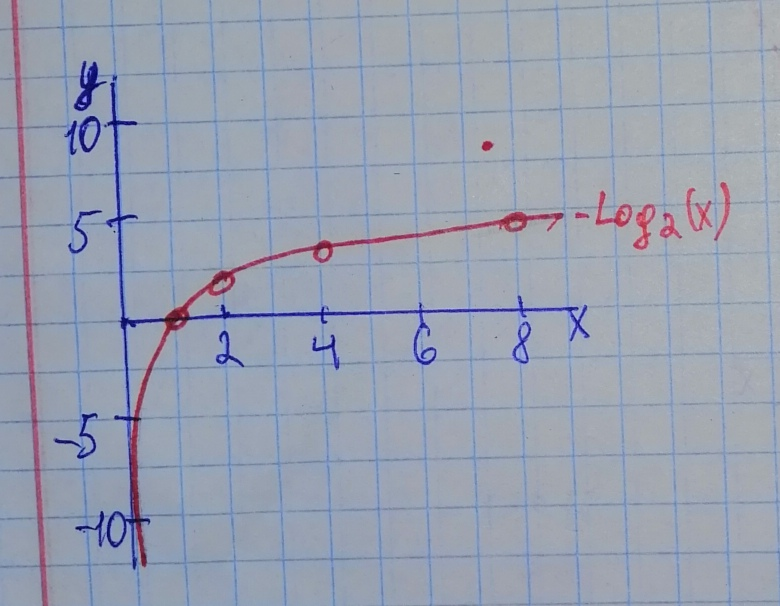# Begin by graphing f(x)=log_{2}x Then use transformations of this graph to graph the given function. What is the vertical asymptote? Use the graphs to determine each function s domain and range. g(x)=frac{1}{2}log_{2}xboitshupoO 2021-01-10 Answered
Begin by graphing
$f\left(x\right)={\mathrm{log}}_{2}x$
Then use transformations of this graph to graph the given function. What is the vertical asymptote? Use the graphs to determine each function s domain and range.
$g\left(x\right)=\frac{1}{2}{\mathrm{log}}_{2}x$
You can still ask an expert for help

• Questions are typically answered in as fast as 30 minutes

Solve your problem for the price of one coffee

• Math expert for every subject
• Pay only if we can solve itodgovoreh

Step 1
For the following Logarithmic function defined by
$f\left(x\right)={\mathrm{log}}_{2}x$
Graphing Logarithmic function given in equation requires setting up table of coordinates, so that

Step 2
We plot the following points between determined from the table of coordinates and connect them with the continuous curve which represent the Logarithmic function $f\left(x\right)={\mathrm{log}}_{2}x$ as shown in Figure (1)
Figure (1) represent the graph of Logarithmic function $f\left(x\right)={\mathrm{log}}_{2}x$Step 3
Note that:
The y-axis which represented by the equation $x=0$ is the vertical asymptote, so that the curve approches, but never touches the positive portion of the y-axis as shown in figure (1).
The domain of $f\left(x\right)={\mathrm{log}}_{2}\left(x\right)$ is all positive real numbers and the range is all real numbers
Step 4
To graph the Logarithmic function $g\left(x\right)=\frac{1}{2}{\mathrm{log}}_{2}\left(x\right)$, we vertically shri
the graph of the function $f\left(x\right)={\mathrm{log}}_{2}\left(x\right)$ because Vertically shri
to $g\left(x\right)=\frac{1}{2}{\mathrm{log}}_{2}\left(x\right)\right)$, then the Logarithmic function $f\left(x\right)={\mathrm{log}}_{2}\left(x<$